# What is Poisson Distribution?

An solid overview of the Poisson distribution, starting from why it is needed, how it stacks up to binomial distribution, deriving its formula mathematically, and more.comments

By Aerin Kim, Microsoft AI Research

Before setting the parameter λ and plugging it into the formula, let’s pause a second and ask a question.

Why did Poisson have to invent the Poisson Distribution?

Why does this distribution exist (= why did he invent this)?

When should Poisson be used for modeling?

### 1. Why did Poisson invent Poisson Distribution?

To predict the # of events occurring in the future!

More formally, to predict the probability of a given number of eventsoccurring in a fixed interval of time.

If you ever sold something, this “event” can be defined, for example, as a customer purchases something from you (the moment of truth, not just browsing). It can be how many visitors you get on your website a day, how many clicks your ads get for the next month, how many phone calls you get during your shift, or even how many people will die from a fatal disease next year, etc.

Below is an example of how I’d use Poisson in real life.

`Every week, on average, 17 people clap for my blog post. I’d like to predict the # of ppl who would clap next week because I get paid weekly by those numbers.What is the probability that exactly 20 people (or 10, 30, 50, etc.) will clap for the blog post next week?`

### 2. For now, let’s assume we don’t know anything about the Poisson Distribution. Then how do we solve this problem?

One way to solve this would be to start with the number of reads. Each person who reads the blog has some probability that they will really like it and clap.

This is a classic job for the binomial distribution since we are calculating the probability of the number of successful events (claps).

A binomial random variable is the number of successes x in n repeated trials. And we assume the probability of success is constant over each trial.

However, here we are given only one piece of information — 17 ppl/week, which is a “rate” (the average # of successes per week, or the expected value of x). We don’t know anything about the clapping probability p, nor the number of blog visitors n.

Therefore, we need a little more information to tackle this problem. What more do we need to frame this probability as a binomial problem? We need two things: the probability of success (claps) p & the number of trials (visitors) n.

Let’s get them from the past data.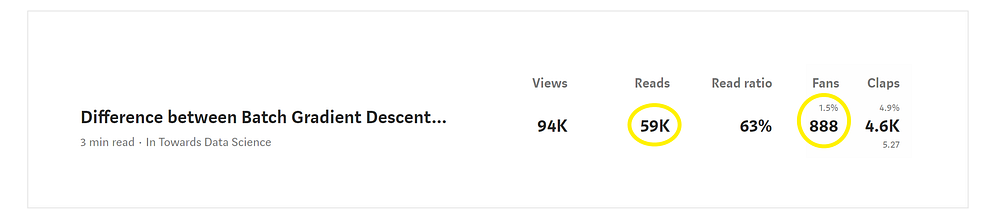These are stats for 1 year. A total of 59k people read my blog. Out of 59k people, 888 of them clapped.

Therefore, the # of people who read my blog per week (n) is 59k/52 = 1134. The # of people who clapped per week (x) is 888/52 =17.

`# of people who read per week (n) = 59k/52 = 1134# of people who clap per week (x) = 888/52 = 17Success probability (p) : 888/59k = 0.015 = 1.5%`

### Using the Binomial PMF, what is the probability thatI’ll get exactly 20 successes (20 people who clap) next week?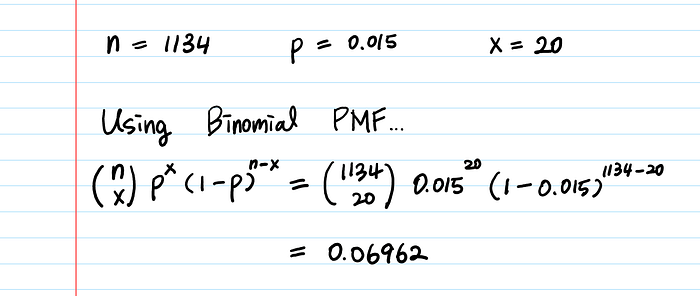```<Binomial Probability for different x’s>
╔══════╦═══════════════════╗
║   x  ║  Binomial P(X=x)  ║
╠══════╬═══════════════════╣
║  10  ║      0.02250      ║
║  17  ║      0.09701      ║  🡒 The average rate has the highest P!
║  20  ║      0.06962      ║  🡒 Nice. 20 is also quite Likely!
║  30  ║      0.00121      ║
║  40  ║    < 0.000001     ║  🡒 Well, I guess I won’t get 40 claps..
╚══════╩═══════════════════╝```

We just solved the problem with a binomial distribution.

Then, what is Poisson for? What are the things that only Poisson can do but Binomial can’t?

### 3. The shortcomings of the Binomial Distribution

a) A binomial random variable is “BI-nary” — 0 or 1.

In the above example, we have 17 ppl/wk who clapped. This means 17/7 = 2.4 people clapped per day, and 17/(7*24) = 0.1 people clapping per hour.

If we model the success probability by hour (0.1 people/hr) using the binomial random variable, this means most of the hours get zero claps but some hours will get exactly 1 clap. However, it is also very possible that certain hour will get more than 1 clap (2, 3, 5 claps, etc.)

The problem with binomial is that it can NOT contain more than 1 event in the unit time (in this case, 1 hr). The unit time can only have 0 or 1 event.

Then, how about dividing 1 hour into 60 minutes, and make unit time smaller, for example, a minute? Then 1 hour can contain multiple events. (Still, one minute will contain exactly one or zero events.)

Is our problem solved now?

Kind of. But what if, during that one minute, we get multiple claps? (i.e. someone shared your blog post on Twitter and the traffic spiked at that minute.) Then what? We can divide a minute into seconds. Then our time unit becomes a second and again a minute can contain multiple events. But this binary container problem will always exist for ever-smaller time units.

The idea is, we can make the Binomial random variable handle multiple events by dividing a unit time into smaller units. By using smaller divisions, we can make the original unit time contain more than one event.

Mathematically, this means n → ∞.

Since we assume the rate is fixed, we must have p → 0. Because otherwise, n*p, which is the number of events, will blow up.

Using the limit, the unit times are now infinitesimal. We no longer have to worry about more than one event occurring within the same unit time. And this is how we derive Poisson distribution.

b) In the Binomial distribution, the # of trials (n) should be known before hand.

If you use Binomial, you cannot calculate the success probability only with the rate (i.e. 17 ppl/week). You need “more info” (& p) in order to use the binomial PMF.

The Poisson Distribution, on the other hand, doesn’t require you to know n nor p. We are assuming n is infinitely large and p is infinitesimal. The only parameter of the Poisson distribution is the rate λ (the expected value of x). In real life, only knowing the rate (i.e., during 2pm~4pm, I received 3 phone calls) is much more common than knowing both n & p.

### 4. Let’s derive the Poisson formula mathematically from the Binomial PMF.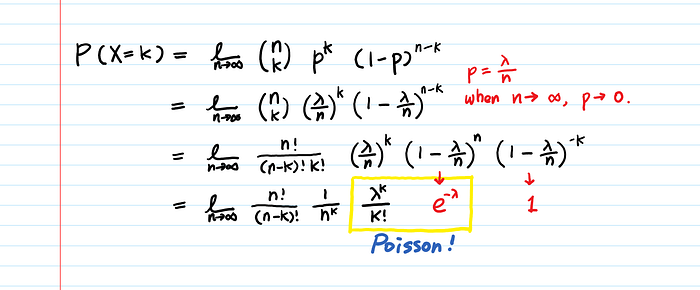Deriving Poisson from Binomial

Now you know where each component λ^k , k! and e^-λ come from!

Finally, we only need to show that the multiplication of the first two terms n!/((n-k)!*n^k) is 1 when n approaches infinity.It is 1.

We got the Poisson Formula!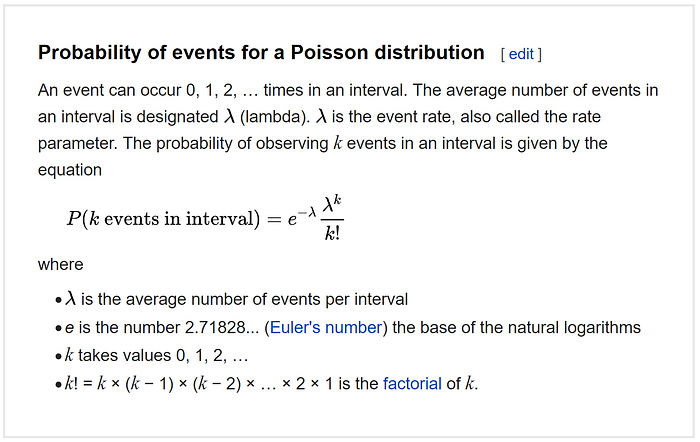Now the Wikipedia explanation starts making sense.

Plug your own data into the formula and see if P(x) makes sense to you!

Below is mine.

```< Comparison between Binomial & Poisson >
╔══════╦═══════════════════╦═══════════════════════╗
║   k  ║  Binomial P(X=k)  ║  Poisson P(X=k;λ=17)  ║
╠══════╬═══════════════════╬═══════════════════════╣
║  10  ║      0.02250      ║        0.02300        ║
║  17  ║      0.09701      ║        0.09628        ║
║  20  ║      0.06962      ║        0.07595        ║
║  30  ║      0.00121      ║        0.00340        ║
║  40  ║    < 0.000001     ║      < 0.000001       ║
╚══════╩═══════════════════╩═══════════════════════╝
* You can calculate both easily here:
Binomial:  https://stattrek.com/online-calculator/binomial.aspx
Poisson :  https://stattrek.com/online-calculator/poisson.aspx```

A few things to note:

1. Even though the Poisson distribution models rare events, the rate λ can be any number. It doesn’t always have to be small.
2. The Poisson Distribution is asymmetric — it is always skewed toward the right. Because it is inhibited by the zero occurrence barrier (there is no such thing as “minus one” clap) on the left and it is unlimited on the other side.
3. As λ becomes bigger, the graph looks more like a normal distribution.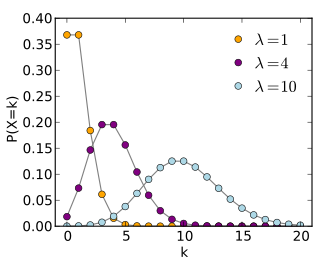1. The Poisson Model Assumptions

a. The average rate of events per unit time is constant.

This means the number of people who visit your blog per hour might not follow a Poisson Distribution, because the hourly rate is not constant (higher rate during the daytime, lower rate during the nighttime). Using monthly rate for consumer/biological data would be just an approximation as well, since the seasonality effect is non-trivial in that domain.

b. Events are independent.

The arrivals of your blog visitors might not always be independent. For example, sometimes a large number of visitors come in a group because someone popular mentioned your blog, or your blog got featured on Medium’s first page, etc. The number of earthquakes per year in a country also might not follow a Poisson Distribution if one large earthquake increases the probability of aftershocks.

5. Relationship between a Poisson and an Exponential distribution

If the number of events per unit time follows a Poisson distribution, then the amount of time between events follows the exponential distribution. The Poisson distribution is discrete and the exponential distribution is continuous, yet the two distributions are closely related.

Let’s go deeper: Exponential Distribution Intuition

Bio: Aerin Kim is a Research Engineer at Microsoft AI Research and this is her notepad for Applied Math / CS / Deep Learning topics. Follow her on Twitter for more!

Original. Reposted with permission.

Related: